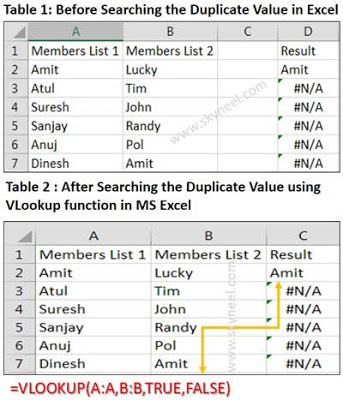# How to Search Duplicate Values with VLookup function

working on a same worksheet then after applying VLookup formula you can easily search duplicate values with VLookup function in MS Excel.

Hi, everyone today we discuss another very important topic which you face when you go for interview during the doing job in any company or facing the questions about “VLookup” during the interview session. You know very well “VLookup” is one of the most important functions which are found in “Lookup and Reference” category of Microsoft Excel. There are lots of different examples of VLookup function in Excel, which you can use search Duplicate Values with VLookup function.

### How to Search duplicate values with VLookup Function in MS Excel:

When we are using “VLookup” function, most of the cases we use “Exact Match” method in MS Excel. Today I try to tell you how to use VLookup” function for searching “Duplicate Values” in range lookup.

#### Definition of VLookup function:

This function is found in the “Lookup and Reference” categories of Microsoft Excel So have a look and take few easily use appropriate match in range lookup. How could you find any duplicate values in a huge database with the help of VLookup function in MS Excel. In that situation, if you don’t give proper answer then you will be rejected for the job during facing the interview session but if you have proper knowledge about the all-important commands and what is the use of VLookup function in MS Excel then you will be able to give exact answer of any query and you will be selected for the job. So, due to watching the importance of ” VLookup” function today, we have to discuss How to find duplicate values using VLookup function in MS Excel in this article.

#### Syntax of VLookup function:

The Microsoft Excel VLookup function searches a value in the extreme left column of the table_array and provides the value in the same row which is based on the index number.

= VLookup (lookup_value, table_array, col_index_num, [range])

Note: Before using the VLookup function, first you need to arrange first column of a table or array in the ascending order, otherwise VLookup function is not able to provide the accurate result.

Lookup_value: The value to be searched in the extreme first column of the table array.

Table_array: Two or more columns of data. Give cell reference of a range / range name.

Col_index_num: The column index_number in the table from which the searched value must be returned.

If col_index_num of returns 1 the value in the first column in table_array.

If col_index_num is: Less than 1, this function returns an error value the #VALUE!.

If it is a greater than the number of columns in table_array, this function returns error value #REF!.

Range_lookup: It is an options part of this function. There are two options available in this part.

TRUE: Appropriate Match: This option work properly when first column of your table will be sorted in ascending order only otherwise it displays wrong
result.

False: Exact Match: VLookup by default check only on exact match method.

For E.g: Have a look and find any duplicate values on the same worksheet during applying VLookup formula in any sorted and unsorted table or database.

#### Steps to Find Duplicate Values with VLookup Function in MS Excel:

Step 1: Create the following table
For E.g.: A1:A7={“Members List 1″,”Amit”,”Atul”,”Suresh”,”Sanjay”,”Anuj”,”Dinesh”}
B1:B7={“Members List 2″,”Lucky”,”Tim”,”John”,”Randy”,”Pol”,”Amit”}

Note: After creating this table, If someone ask you find out the duplicate value in both range which is given on the same worksheet using VLookup function in MS Excel. Notice that both ranges have a common name “Amit” and now watch the process how to found this duplicate value using VLookup function in same worksheet.

Step 2: Take the cursor on a particular location where you want to view the result after applying the VLookup function. For E.g. type Result in the cell C1.Step 3: Now, take cursor on cell C2 and type the following given formula to searching duplicate value using VLookup function on same worksheet in MS Excel:

=VLOOKUP(A:A,B:B,TRUE,FALSE)

after typing the formula press enter or return key, MS Excel shows you duplicate value “Amit” in cell C2 which is found in both range A1:A7 and B1:B7 using the VLookup function on the same worksheet.

Step 4: Again select the cell C2 and drag the fill handle up to cell C7 and release the mouse pointer. Now, you will get rest of the cells shows #N/A as a result because there is no another duplicate value in both range of cells, but if you make any kind of modification in the second range of B1:B7 your answer will be changed.

I hope you like How to Search Duplicate Values with VLookup function guide, Please give your important suggestion regarding this article in the comment box and click on the like or share button to help others who are facing problem for Search Duplicate Values with VLookup function in MS Excel. Thanks to all.